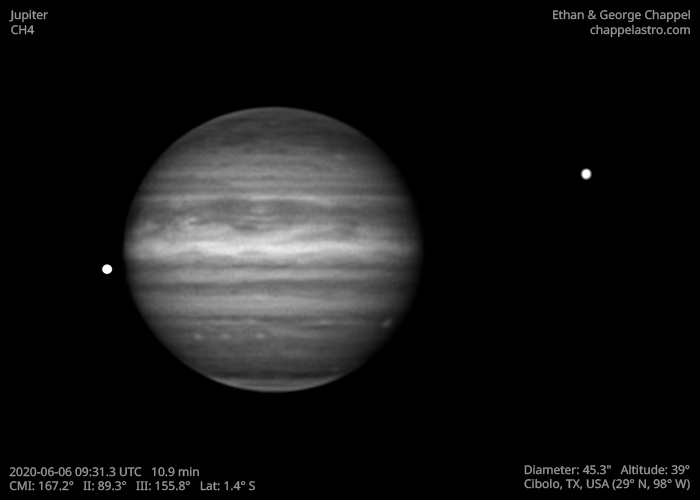# Jupiter 2020-06-06 09:31 UTC

CM1: 167.20°

CM2: 89.30°

CM3: 155.80°

Description

Impressive seeing and good transparency. This was the first night with my new laptop, a Dell Precision 7540. This laptop is much more upgradable than my late-2013 MacBook Pro, where the limited drive space would force me to stop imaging and move data off.

Notes:

• Oval BA rotating off the day side.
• Io has just moved out from behind Jupiter while Callisto also moves away

Equipment

ZWO ASI290MM

Celestron EdgeHD 14

Logs
```FireCapture v2.6  Settings
------------------------------------
Observer=Ethan Chappel
Location=Cibolo
Scope=Celestron C14 EdgeHD
Camera=ZWO ASI290MM
Filter=CH4
Profile=Jupiter
Diameter=45.22"
Magnitude=-2.60
CMI=168.7° CMII=90.8° CMIII=157.3°  (during mid of capture)
FocalLength=3400mm (F/9)
Resolution=0.18"
Filename=2020-06-06-0934_3-EC-CH4-Jup.ser
Date=060620
Start=093152.288
Mid=093422.293
End=093652.298
Start(UT)=093152.288
Mid(UT)=093422.293
End(UT)=093652.298
Duration=300.010s
Date_format=ddMMyy
Time_format=HHmmss
LT=UT -6h
Frames captured=5000
File type=SER
Binning=no
ROI=752x394
ROI(Offset)=0x0
FPS (avg.)=16
Shutter=60.00ms
Gain=420 (70%)
HighSpeed=on
AutoGain=off
USBTraffic=100
SoftwareGain=10 (off)
FPS=100 (off)
Brightness=1 (off)
Gamma=50 (off)
AutoExposure=off
AutoHisto=75 (off)
Histogramm(min)=0
Histogramm(max)=111
Histogramm=43%
Noise(avg.deviation)=4.26
Limit=300 Seconds
Sensor temperature=30.8°C
Focuser position=810
FireCapture v2.6  Settings
------------------------------------
Observer=Ethan Chappel
Location=Cibolo
Scope=Celestron C14 EdgeHD
Camera=ZWO ASI290MM
Filter=CH4
Profile=Jupiter
Diameter=45.22"
Magnitude=-2.60
CMI=165.1° CMII=87.3° CMIII=153.8°  (during mid of capture)
FocalLength=3350mm (F/9)
Resolution=0.18"
Filename=2020-06-06-0928_4-EC-CH4-Jup.ser
Date=060620
Start=092559.169
Mid=092829.179
End=093059.190
Start(UT)=092559.169
Mid(UT)=092829.179
End(UT)=093059.190
Duration=300.021s
Date_format=ddMMyy
Time_format=HHmmss
LT=UT -6h
Frames captured=5001
File type=SER
Binning=no
ROI=752x394
ROI(Offset)=0x0
FPS (avg.)=16
Shutter=60.00ms
Gain=420 (70%)
HighSpeed=on
AutoGain=off
USBTraffic=100
SoftwareGain=10 (off)
FPS=100 (off)
Brightness=1 (off)
Gamma=50 (off)
AutoExposure=off
AutoHisto=75 (off)
Histogramm(min)=0
Histogramm(max)=100
Histogramm=39%
Noise(avg.deviation)=4.23
Limit=300 Seconds
Sensor temperature=31.0°C
Focuser position=810
```